Negacyclic Convolution Using

Polynomial Transforms on Hypercubes

Barry Fagin

Thayer School of Engineering

Dartmouth College

Hanover, NH 03755

603-646-3060

barry.fagin@dartmouth.edu

EDICS classification: 4.1.1, 4.1.2, 7.1.1

ABSTRACT

We present a polynomial transform based algorithm for calculating products modulo zn+1 on a hypercube. All interprocessor communication in this algorithm occurs over a Hamming Distance of 1; that is, processors communicate only with their immediate neighbors. We have implemented this algorithm on a Connection Machine, and discuss our performance results. Current figures show a time of 358 msec for negacyclic convolution of 1K 16-bit samples, up to about 8 seconds seconds for a 64K data set. We discuss the use of this algorithm in the calculation of convolution, compare communication costs with the FFT, and discuss directions for future work.

Permission to publish this abstract separately is granted.

1.0 Introduction

In , Nussbaumer proposed the polynomial transform algorithm for the computation of negacylic convolution (polynomial products modulo zn+1). Polynomial transforms can be used to calculate circular convolutions with a number of additions and multiplications comparable to the FFT, without the use of trigonometric functions or complex arithmetic. Since they employ integer arithmetic exclusively, polynomial transforms may be used to calculate convolutions to a greater degree of precision more easily than with conventional techniques. If sufficient memory is available, exact convolution can be calculated.

In addition to their arithmetic advantages, polynomial transforms can be used to efficiently map multidimensional convolutions onto one dimensional convolutions, or to compute multidimensional convolutions directly. Perhaps most importantly, polynomial transforms have an FFT-like structure, lending themselves to efficient implementation on both single and multiple processors.

FFT-like algorithms can be implemented in parallel on a hypercube, a multiprocessor interconnection network . A hypercube contains 2n processors, each of which is connected to n other processors. Each processor P has an n-bit address A; the addresses of the neighbors of P are just those addresses that differ from A in exactly one bit. The Hamming Distance between two processors U and V is the sum of the bitwise exclusive or of their addresses. This value represents the number of links in the network that must be traversed for processor U to communicate with processor V. We will refer to interprocessor communication with a Hamming Distance of 1 as "nearest neighbor" communication.

Johnsson and Ho have studied the computation of the FFT on hypercubes , noting the importance of utilizing the full bandwidth of the interconnection network. For the FFT and related algorithms, interprocessor communication is the principal factor affecting performance.

In this paper, we present an algorithm for the calculation of the polynomial transform on a hypercube. Despite the unusual data permutations and access patterns required by the polynomial transform, all interprocessor communication in our algorithm occurs over a Hamming distance of 1. In other words, all processors communicate exclusively with their immediate neighbors in the cube.

We begin with the complete Nussbaumer Negacyclic Convolution Algorithm, of which polynomial transforms are the central component. We also discuss how this procedure can be used to calculate true convolution. We then show how to eliminate the data permutation suggested by a naive implementation, and discuss how to calculate polynomial transforms on a hypercube. Finally, we analyze the performance of the algorithm on the Connection Machine, a commercially available hypercube multiprocessor, and compare the Nussbaumer algorithm for convolution with the FFT. We conclude with a discussion of directions for future work.

2.0 The Nussbaumer Algorithms for Negacyclic Convolution and Integer Convolution

This section explains the Nussbaumer algorithms for negacyclic convolution and convolution, and recapitulates material found in . Readers familiar with these algorithms may skip to section 3.0.

Let A(Z), B(Z) be polynomials of length L=2v. We wish to calculate the coefficients of the polynomial C(Z) defined by:

L-1

C(Z) = cpZp A(Z)B(Z) modulo ZL+1 (1)

p=0

where

L-1 L-1

A(Z) = anZn, B(Z) = bmZm (2)

n=0 m=0

We define L=L1*L2, L1=2l1, and L2=2l2, and introduce the new indices n1, n2, m1, and m2 such that n = L1n2 + n1, m = L1m2 + m1, where mi and ni run from 0 through Li-1. If we define a new variable Z1 = ZL1, we may rewrite the expressions in equations 1 and 2 as

L1-1 L2-1

A(Z,Z1) = ∑ ∑ aL1n2+n1 Zn1Z1n2

n1=0 n2=0

L1-1 L2-1

B(Z,Z1) = ∑ ∑ bL1m2+m1 Zm1Z1m2

m1=0 m2=0

C(Z,Z1) A(Z,Z1) B(Z,Z1) modulo (ZL1-Z1), (Z1L2+1) (3)

In this form, the polynomial C becomes a product of polynomials in Z modulo ZL1-Z1. This, in turn is the cyclic convolution of the polynomials An1 and Bm1 defined below:

L2-1

An1(Z1) = aL1n2+n1 Z1n2

n2=0

L2-1

Bm1(Z1) = bL1m2+m1 Z1m2 (4)

m2=0

Once we have calculated this convolution, we may obtain C(Z,Z1), and hence C(Z), by reducing the convolution modulo ZL1-Z1.

Nussbaumer  has shown that the calculation of (3) may be performed very efficiently with the use of polynomial transforms. We define the polynomial transforms of (4) as

2L1-1

A'k(Z1) ∫An1(Z1)Z1(L2/L1)n1k modulo (Z1L2+1)

n1=0

k = 0 ... 2L1-1

and

2L1-1

B'k(Z1) ∫Bm1(Z1)Z1(L2/L1)m1k modulo (Z1L2+1) (5)

m1=0

k = 0 ... 2L1-1

with An1(Z1)=0 for n1 >= L1, Bm1(Z1)=0 for m1 >= L1.

To compute the desired convolution, we calculate the polynomial transforms defined above, and then calculate the k point-by-point polynomial products modulo Z1L2+1 recursively. The recursion halts with L2=2, in which case direct multiplication in the ring z2+1 is performed.

We may then calculate the convolution by taking the inverse polynomial transform of the product A'k(Z1)*B'k(Z1):

2L1-1

Cp(Z1) = (1/2L1) A'k(Z1)*B'k(Z1)Z1-(L2/L1)p1k mod (Z1L2+1)

k=0

p=0 ... 2L1-1 (6)

The calculation of forward polynomial transforms can be viewed geometrically as a succession of folding and splitting operations. A 1 x 2n vector is transformed into a 2l1+1 x 2l2 array, where n = l1+l2, l1 as large as possible but less than or equal to l2. This is followed by the calculation of a polynomial transform, repeated recursively for each row of the array until the array is of dimension 2n+Èlog n¢-1 x 2. Polynomial multiplication modulo z2+1 is performed, followed by an inverse polynomial transform and a "collapsing" phase, corresponding to the reduction modulo ZL1-Z1. This is repeated recursively until the 1 x 2n negacyclic convolution of the input vectors is obtained.

Polynomial transforms possess the convolution property because Z1 is a primitive 2L2th root of unity in the ring of polynomials modulo Z1L2+1. No multiplications are needed in polynomial transform calculation, as multiplication by a power of Z1 is equivalent to a rotation and inversion of the target vector.

Cyclic convolution is equivalent to polynomial multiplication modulo zn-1. For n a power of two, we note that zn-1 factors into zn/2+1 and zn/2-1, zn/2-1 factors into zn/4+1 and zn/4-1, and so on to z+1 and z-1. Since these polynomials are irreducible over the rationals, we may use polynomial transforms to calculate negacylic convolution, and then combine products using the Chinese Remainder Theorem to compute true convolution.

The reductions are all accomplished via 2(N-1) additions and subtractions; no multiplications are necessary . If A(Z) and B(Z) denote polynomial products modulo zm/2+1 and zm/2-1 respectively, the Chinese Remainder construction for their product modulo zm-1 is given by

P(Z) = ((1-Zm/2)A(Z) + (1+Zm/2)B(Z))/2

This requires m additions, and no multiplications . Thus the computationally significant portion of the convolution calculation is the determination of negacyclic convolution products. We shall focus, therefore, on the efficient calculation of negacyclic convolution.

Finally, we note that the polynomial transform and the FFT bear a strong similarity to one another. The two algorithms have an identical computational structure; only the basic elements and operations differ. The FFT manipulates complex scalars in the ring of complex numbers, while the polynomial transform manipulates polynomials in the ring of polynomials modulo zN+1. Both techniques employ log N iterations of the familiar butterfly algorithm. Both algorithms employ multiplications of a primitive Nth root of unity. In the FFT, this corresponds to a complex multiplication. In the polynomial transform, however, this corresponds to a rotation and inversion of a vector. No multiplication is necessary.

3.0 Eliminating Data Permutation

A naive implementation of negacyclic convolution on a multiprocessor would directly manipulate a two-dimensional array, rearranging data as necessary to achieve the reordering operations required by changes in transform length and vector size. We will see in this section that no data rearrangement is necessary. By a careful analysis of the permutation operations, we will show that data rearrangement affects only two quantities relevant to the polynomial transform. By parametrizing the polynomial transform algorithm to include these quantities, we may calculate negacyclic convolution without the data permutation suggested by a naive implementation of the algorithm on a multiprocessor.

We begin by examining how a single sequence may be stored on a hypercube so that minimal permutation is necessary to compute its polynomial transform for negacyclic convolution. We will list the contents of the hypercube in linear decreasing order. In other words, the sequence (Pj-1, Pj-2, ... , P0) describes a hypercube with value Pj-1 at node j-1, Pj-2 at node j-2, and so forth. If the number of nodes is greater than the number of elements in the sequence, all nodes not listed are assumed to be zero. In this configuration, an interprocessor communication between two processors with addresses that differ only in bit position j corresponds to nearest-neighbor communication along dimension j of the hypercube.

We examine two quantities of interest, the log of the size of the nonzero data set, denoted by s, and the log of the vector separation distance, denoted by d. The vector separation distance is the number of intervening processors between elements of a vector on a linearly ordered hypercube. For example, if the vectors [7 5 3 1] and [6 4 2 0] were stored on a hypercube as (7 5 3 1 6 4 2 0), the vector separation distance would be 0. If the vectors were stored as (7 6 5 4 3 2 1 0), the distance would be 1. In the polynomial transform algorithm, the vector separation distance is always greater than or equal to 1.

Consider the initial input sequence (xn-1, xn-2, ... , x0), l1 = În/2˚l2 = Èn/2¢. We first pad it with zeros, giving (0, 0, ... , 0, xn-1, xn-2, ... , x0). We then have s = log n, d = 0. The polynomial transform can be divided in half, with vectors in each half separated by log distance d. The log of the size of each half is just s, while the total size of the transform is 2l1+1. Each iteration of the Nussbaumer algorithm increases the log separation distance by l1, while doubling the size of the nonzero data set. An example for an initial data set of 8 elements is shown in Figure 1.

We note that if the input data are stored in linear order on the hypercube, then at any time before a transform is calculated the address of a processor can be broken down as shown in Figure 2. The least significant d bits indicate the number of the transform, the next l1 bits indicate the index of the processor's vector within its half transform, the next l2 bits indicate the index of the processor's element within its vector, and the most significant bit indicates which half of the transform contains this processor's vector. Thus the index of an element within a vector can be obtained directly from the address of a processor, while the index of a vector within a transform can be obtained by concatenating the most significant bit with bits d+l1-1 through d of the processor address.

4.0 Polynomial Transforms on the Hypercube

We now show how to calculate polynomial transforms efficiently using nearest-neighbor communication on a hypercube. Most of the communication required is associated with the rotation of vectors, corresponding to multiplication by a power of z in the ring zm+1. We show how to calculate a polynomial transform using O(lg3 n) nearest-neighbor communication steps.

The basic operation of the polynomial transform is the butterfly operation

A <- A + RI(B,tw)

B <- A - RI(B,tw)

where A and B are vectors of length 2l2, RI indicates a rotate-and-invert operator, and tw is the twiddle factor denoting the length of the rotation. A and B are updated in parallel. Since the distance between vectors in a polynomial transform butterfly is always a power of two, and since elements in a vector are always equidistant from one another, the interprocessor communication associated with the addition and subtraction can occur in one nearest-neighbor communication step. We turn our attention, then, to rotation and inversion.

To accomplish the rotation and inversion, we break each butterfly step of the polynomial transform into a succession of rotations of powers of two. For example, if we have eight butterflies with the twiddle factors (6,4,2,0,6,4,2,0), we may accomplish the rotations as (4,4,0,0,4,4,0,0) and (2,0,2,0,2,0,2,0). So if we can show how to rotate a sequence by an arbitrary power of two using nearest-neighbor communication, we can calculate the polynomial transform. (Inversion is simply the parallel subtraction of selected values from zero, requiring no interprocessor communication).

Rotation of sequences by a power of two on a hypercube is in fact quite simple. Consider a sequence X of length 2n. We may rotate it to the left 2m positions in the following way:

FOR k = m to n-1

IF k > m

enable processors X[i] with bits k-1 through m of i = 0 (= 1 if rotation is to the right)

ELSE

enable all processors

all enabled processors swap elements along axis k

Examples of rotation are shown in Figure 3.

Since we can rotate by an arbitrary power of two using only nearest-neighbor communication, we can calculate a polynomial transform using only nearest-neighbor communication. The technique for the inverse polynomial transform is similar.

5.0 Analysis

We now consider hardware and time requirements of the negacyclic convolution algorithm.

5.1 Processor Requirements

The number of processors required for this algorithm can be calculated as follows. Let N be the size of the input sequences, let n = log N. Initially, we compute a 2(l1+1) x 2l2 transform. We then compute 2(l1+1) products of length 2l2 recursively. Each of these require the computation of a 2(l1'+1) x 2l2' transform, where l1' = Îl2/2˚l2' = Èl2/2¢. The process then repeats until l2 = 1.

For n > 0, we define the splitting tree rooted at n as follows:

1) The root of the splitting tree rooted at n is a node with value n.

2) If n > 1, the left child of the root of the splitting tree rooted at n is a node with value În/2˚and the right child is the splitting tree rooted at Èn/2¢. If n = 1, there are no children.

We obtain the augmented splitting tree rooted at n by adding 1 to all left children in the splitting tree rooted at n. The splitting and augmented splitting trees root at n=18 are shown in Figure 4.

We see that the log of the number of processors required for calculating the negacyclic convolution of two sequences of length N = 2n is just the sum of the leaves of the augmented splitting tree. For the previous example, computing the negacyclic convolution of two sequences of length 218 requires computing 210 polynomial products of length 29, each of which requires computing 25 polynomial products of length 25, and so forth. Since the leaves represent the log of the number of products, we sum the leaves to obtain the log of the number of processors required.

A simple inductive argument will show that the sum of the leaves of the splitting tree rooted at n is just n, and that the number of left children is Èlog n¢. Thus the sum of the leaves of the augmented splitting tree rooted at n is n + Èlog n¢. This gives the log of number of processors required by the negacyclic convolution algorithm as log N + Èlog log N¢, where N is the length of the input sequences. We assume for convenience that N is a power of two. We have, therefore

P = 2(log N + Èlog log N¢)

where P is the number of processors. It is not difficult to show that N log N <= P < 2Nlog N.

It is also possible to keep the number of processors equal to twice the size of the original data set, by repeatedly copying newly created data values from the upper half of the processor set to the lower half, and clearing the upper half for the next iteration. This keeps the number of processors constant while doubling the amount of work each processor must perform with each iteration. We used this technique in our implementation of negacyclic convolution, described in section 6.0. In this case the previous analysis calculates the memory requirements of the algorithm; P is now the number of digits.

5.2 Communication Requirements

We may calculate the number of communication steps required as follows. For a single transform, butterfly iteration i (numbered from 0) requires 0 + 1 + ... + i = i(i+1)/2 communication steps for rotation alone. Thus the total number of steps for rotation is

l1

i(i+1)/2 = (l13 + 3l12 + 2l1)/6

i=1

Each iteration also requires one step for the butterfly operation, so the total number of steps for one transform is (l13 + 3l12 + 2l1)/6 + l1 + 1. Since l1 + 1 is the log of the transform size, the number of steps needed to calculate a polynomial transform of size N is O(lg3 N). Each l1/l2 pair has three transforms and one collapse operation associated with it. The collapse stage requires l2 steps to rotate the sequences by one position, plus one step to compute the sums. Thus the number of steps required for a single l1/l2 pair is

STEPS(l1,l2) = 3[(l13 + 3l12 + 2l1)/6 + l1 + 1] + l2 + 1

We may calculate the number of steps required for inputs of size N = 2n by splitting n into l1 and l2, calculating STEPS(l1,l2), adding it to the total, setting n = l2, and repeating until n = 1. We then add two for the steps needed by point-by-point multiplication.

5.3 The Elimination of Rotation and Inversion

Many hypercubes support arbitrary interprocessor communication. That is, any processor can communicate with any other processor in a fixed number of cycles. This means that rotation and inversion are not strictly necessary. If for any processor we know the number of the butterfly, the twiddle factor, and the quantities s and d, we can compute the address required for interprocessor communication. This would eliminate the steps associated with rotation in the polynomial transform. A polynomial transform of length 2l1+1 could then be calculated in l1+1 communication steps, instead of (l13 + 3l12 + 2l1)/6 +l1 + 1.

Which type of communication is appropriate depends on the differences in speed between them. We will see in the next section why we chose nearest-neighbor communication.

6.0 Performance Results

We have implemented the negacyclic convolution algorithm on the Connection Machine, a hypercube multiprocessor. The results presented here were obtained on a CM with 8K processors, each connected to 64K of memory.

The CM supports virtual processors, a software abstraction that permits the user to write programs as if more processors were present than those physically available. Using virtual processors permits the user to work with data sets larger than the number of processors on the machine she is using, with a corresponding increase in execution time. When the number of processors required is greater than the number physically available, the system is said to be saturated. For the results shown here, saturation occurs when N = 212. For more information on virtual processors, the reader is referred to .

The CM supports two kinds of interprocessor communication: NEWS grid communication, of which nearest-neighbor communication is a special case, and arbitrary communication. Arbitrary communication, while much more flexible, is about 400 times slower than nearest-neighbor communication. Thus a nearest-neighbor based polynomial transform algorithm will outperform an arbitrary-communication based one on the Connection Machine for all l1 such that

(l13 + 3l12 + 2l1)/6 + l1 + 1 < 400(l1+1)

or l1 ª 48. This corresponds to input sizes much larger than the capacity of the Connection Machine. Thus for all feasible inputs on a Connection Machine, a nearest-neighbor based algorithm will outperform an arbitrary-communication based one. This is typical of CM programming: nearest-neighbor communication should be used whenever possible.

6.1 Execution Times for Negacyclic Convolution

The performance of the negacyclic convolution algorithm on an 8K Connection Machine is shown below. Figure 5 shows a graph of log execution time versus log sequence length. Graphs for 8, 16, 32, and 64-bit samples are shown.

We see that once saturation occurs (log N = 13), execution time grows linearly with input size. Missing data points for larger values of log N indicate memory requirements that exceed the capabilities of an 8K CM. CM's with more physical processors available will produce correspondingly lower execution times. Running the negacyclic convolution algorithm on a 64K machine, for example, would increase the saturation point to log N = 16, with a corresponding decrease in execution times for larger sequence lengths. A 64K CM would also increase the maximum sequence length to 219.

Sequences of virtually arbitrary size can be processed by the program if the requirement that the input sequence reside in memory is relaxed. CM I/O devices may then be used to transfer portions of the sequence in and out of memory as needed.

6.2 Breakdown of Execution Time

We consider the breakdown of execution time for the results shown above. We divide execution into three categories: communication, multiplication, and other activities. Communication includes all interprocessor communication in all phases of the algorithm. Multiplication includes the time spent in the point-by-point multiplication of the base case, while other activities include local CM computation and front-end overhead. The relative proportions of execution time for the results plotted in Figure 5 are shown in Figure 6.

As expected, interprocessor communication dominates, taking between 52% and 70% of CPU cycles. These numbers indicate that purely computational optimizations, such as using clever techniques to improve multiplication speed, will be only marginally effective. These results confirm the work reported in , in which the importance of effective interprocessor communication is emphasized.

7.0 Comparison With FFT

The algorithm of 2.1 provides an interesting contrast to the more traditional FFT-based approach for the calculation of true convolution. Nussbaumer has analyzed the complexity of this algorithm in terms of the number of additions and multiplications . This measure of complexity is of interest for uniprocessors, but is of limited utility for multiprocessor systems. We consider instead an analysis of communication complexity, a metric better suited to massively parallel systems.

Each reduction requires only one communication step, since the vector of coefficients of a polynomial in z of degree n reduced modulo zn/2 ±1 may be obtained by adding or subtracting the upper and lower halves of the coefficient vector from one another. The CRT calculations also require a single communication step: the upper half of the output polynomial is the sum of the input vectors, while the lower half is the difference. The communication requirements of polynomial product calculation modulo zm+1 were analyzed previously.

Let us assume a SIMD architecture, in which identical operations are performed on all processors concurrently. We first perform the reductions modulo zm ± 1, then the polynomial multiplications, followed by the Chinese Remainder reductions. For an input sequence of length N=2n, the reductions will require n communication steps, as will the Chinese Remainder calculations. Let C+(N) and C-(N) denote the number of communication steps required for the calculation of true and negacyclic convolutions of length N using the Nussbaumer algorithms. We have, then,

C+(N) = C-(N/2) + 2 log N (7)

We note that although we have chosen a different complexity measure from , we reach a similar conclusion: the computationally significant portion of the polynomial transform-based true convolution algorithm is the calculation of negacyclic convolution products.

The number of communication steps for the Nussbaumer algorithm and the traditional FFT-based algorithm is shown in Table 1. For a standard parallel FFT, the number of communication steps is equal to the number of butterflies. Thus the number of communication steps for a standard FFT-based convolution algorithm is 3 log N, as the algorithm requires two forward transforms and an inverse transform.

We see that the communication requirements of the Nussbaumer algorithm are considerably higher, due to the large number of steps required to rotate vector elements in a butterfly operation. This suggests that polynomial transform based methods should be employed on a massively parallel processor only when their unique advantages are required to solve the problem. Typical cases include the multiplication of large integers, or the computation of exact convolution. For convolution problems in which speed is more important than exact results, the FFT is clearly the superior algorithm.

8.0 Conclusions

We have successfully designed and implemented an algorithm for negacyclic convolution on a hypercube, following the polynomial transform method of Nussbaumer. The algorithm employs interprocessor communication with a maximum Hamming distance of 1, the fastest possible on hypercubes. For 16-bit samples, execution times range from 358 msec for negacyclic convolution of a 1K data set, up to about 8 seconds for a 64K data set. Interprocessor communication is the largest single consumer of CPU cycles, taking between 52% and 70% of execution time.

We have compared the communication complexities of the Nussbaumer algorithm for true convolution and the FFT on a massively parallel processor. Our results indicate that the Nussbaumer algorithm should only be employed in those areas where arbitrary precision and exact calculation are required. For most common convolution problems, the FFT remains the better technique. We note, however, that this assumes that the ratio of interprocessor communication time to scalar multiplication time for existing processors will remain constant. Should it decrease, techniques like the polynomial transform that tradeoff multiplications for communication steps may become more attractive.

We are working on several extensions of this work. The present implementation of the algorithm is not optimized for memory usage; careful recoding may permit the convolution of larger data sets. Execution times may be further improved by going to larger sample sizes, breaking up large digits into pieces small enough to be multiplied on the Connection Machine. Converting the samples to floating point numbers and using the CM floating point chips for multiplication could reduce execution time somewhat, although performance will remain limited by interprocessor communication time. Finally, CM I/O devices could be employed to increase the sequence length beyond the limit of 219 of the current implementation.

One promising extension of this work is the multiplication of large integers. Since negacyclic convolution is equivalent to cyclic convolution if the upper halves of the input sequences are zero, the algorithm presented here may be used to obtain the convolution product of large integers as suggested by Knuth . The Nussbaumer algorithm is particularly well suited to the multiplication of large integers because it uses integer arithmetic; if sufficient memory is available, exact answers are easily obtained. We are currently modifying the algorithm to multiply large integers, and expect results shortly.

9.0 Acknowledgements

The results reported here were obtained using the Connection Machine Network Server Pilot Facility, supported under the terms of DARPA contract number DACA76-88-C-0012 05/24/88 and made available to the author by Thinking Machines Corporation. Special thanks are due to TMC employees David Ray, David Gingold, Pat Hickey, and Mark Bromley for their patient assistance to the author.

The author can be reached at the Thayer School of Engineering, Dartmouth College, Hanover NH 03755, or electronically at barry.fagin@dartmouth.edu.

10.0 References

 Jamieson, Leah et. al., "FFT Algorithms for SIMD Parallel Processing Systems", Journal of Parallel and Distributed Computing, Volume 3, No. 1, pp 48-71, March 1986.

 Johnsson, S. et al., "Computing Fast Fourier Transforms on Boolean Cubes and Related Networks", Technical Report NA87-3, Thinking Machines Corporation, Cambridge, Mass.

 Johnsson, S. and Ho, Ching-Tien, "Optimum Broadcasting and Personalized Communication in Hypercubes", IEEE Transactions on Computers, Vol. 38, No. 9, September 1989.

 Knuth, Donald, The Art of Computer Programming, Vol. 2, Addison-Wesley, Reading, Mass, 1981.

 Nussbaumer, Henri "Computation of Convolutions and Discrete Fourier Transforms By Polynomial Transforms", IBM Journal of Research and Development, Vol. 22, pp 134-144, March 1978.

 Nussbaumer, Henri "Fast Polynomial Transform Algorithms for Digital Convolution", IEEE Transactions on Acoustics, Speech, and Signal Processing, Vol. ASSP-28, No. 2, April 1980.

 Connection Machine Technical Summary, Thinking Machines Corporation, Cambridge Massachusetts,1989.

 C/Paris 5.1 User's Manual, Thinking Machines Corporation, Cambridge Massachusetts, 1988.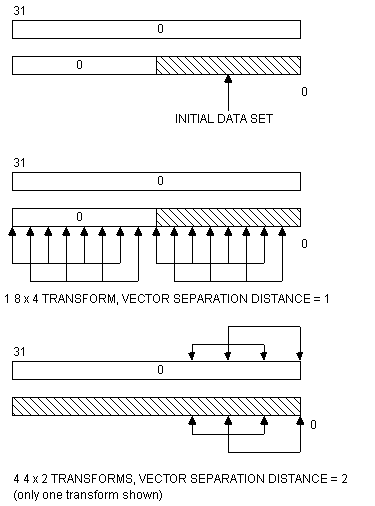Figure 1

In-Place Polynomial Transform Calculation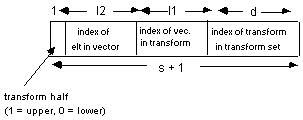Figure 2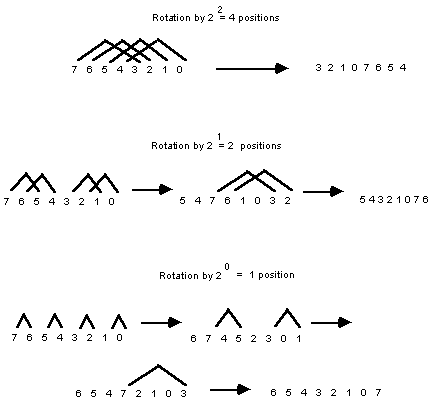Figure 3

Sequence Rotation By a Power of Two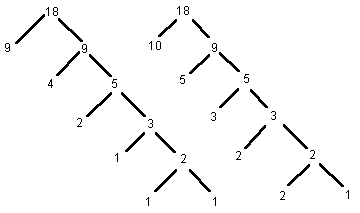Figure 4

Splitting and Augmented Splitting Trees For n=18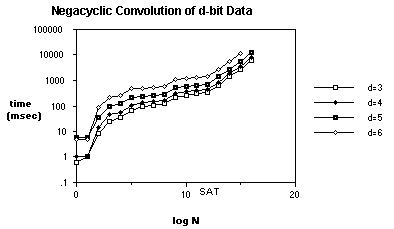Figure 5

Negacyclic Convolution of d-bit Data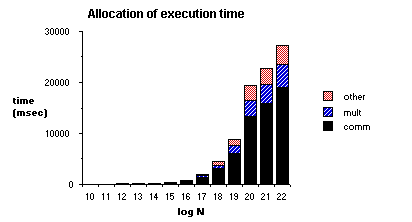Figure 6

Analysis of Execution Time

Table 1

Communication Requirements for

Nussbaumer Algorithm and FFT

n = log N Nuss. FFT

5 47 15

6 62 18

7 85 21

8 96 24

9 135 27

10 151 30

11 201 33

12 225 36

13 293 39

14 309 42

15 398 45

16 434 48

17 547 51

18 564 54

19 704 57

20 755 60

FIGURE AND TABLE CAPTIONS

Figure 1 In-Place Polynomial Transform Calculation

Figure 2 Subfields of Processor Address

Figure 3 Sequence Rotation By a Power of Two

Figure 4 Splitting and Augmented Splitting Trees For n=18

Figure 5 Negacyclic Convolution of d-bit Data

Figure 6 Analysis of Execution Time

Table 1 Communication Requirements for Nussbaumer Algorithm and FFT